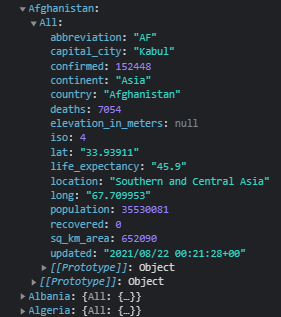# Iterating through and finding values of nested JavaScript objects

How do iterate through the following object and get the value of `confirmed`?I want to add the values together. This is what I’ve tried but both ways are undefined:

``````    for (let i = 0; i < objectSize; i++) {
sumOfCases = sumOfCases + data[i].All.confirmed;
sumOfDeaths = sumOfDeaths + data[i].deaths;
}``````

There are several different ways you could do this, but here’s an option.

``````// assuming your object is defined as data

let sumOfCases = 0;
let sumOfDeaths = 0;
Object.values(data).forEach(country => {
sumOfCases = sumOfCases + country.All.confirmed;
sumOfDeaths = sumOfDeaths + country.All.deaths;
});
``````

Here’s a relevant challenge from the curriculum.

Perfect! Thank you @colinthornton !

1 Like

This topic was automatically closed 182 days after the last reply. New replies are no longer allowed.